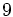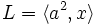# Characteristic not implies fully invariant in class three maximal class p-group

## Statement

Let$p$ be any prime number. There exists a$p$-group that is of class three that is a Maximal class group (?) with a Characteristic subgroup (?) that is not fully invariant.

## Proof

### For odd primes

Suppose$p$ is an odd prime. Let$K$ be the wreath product of groups of order p and$G$ be$K/[[[K,K],K],K]$.$G$ is thus a semidirect product of an elementary abelian group of order$p^3$ and a cyclic group of order$p$. Note that for$p > 3$,$G$ has exponent$p$ but for$p = 3$,$G$ has exponent$9$.

Consider$H = C_G([G,G])$, the centralizer of commutator subgroup.$H$ is a characteristic subgroup. Consider now an element$x$ of order$p$ outside$H$ (such an element exists because of the way the group is defined as a semidirect product) and a subgroup$L$ of$G$ of index$p$ other than$H$, that does not contain$x$. Consider the retraction from$G$ to$\langle x \rangle$ with kernel$L$. This retraction doesn't preserve$H$, so$H$ is not fully invariant.

### For the prime two

Further information: dihedral group:D16

Consider the dihedral group:$G := \langle a,x \mid a^8 = x^2 = e, xax = a^{-1} \rangle$.

Let$H = \langle a \rangle$. Then,$H = C_G([G,G])$, so$H$ is characteristic in$G$. Consider now the subgroup$L = \langle a^2, x \rangle$ and the element$ax$. Consider the retraction to the subgroup$\langle ax \rangle$ with kernel$L$.$H$ is not preserved under this retraction (for instance,$a$ gets mapped to$x$), so it is not fully invariant.